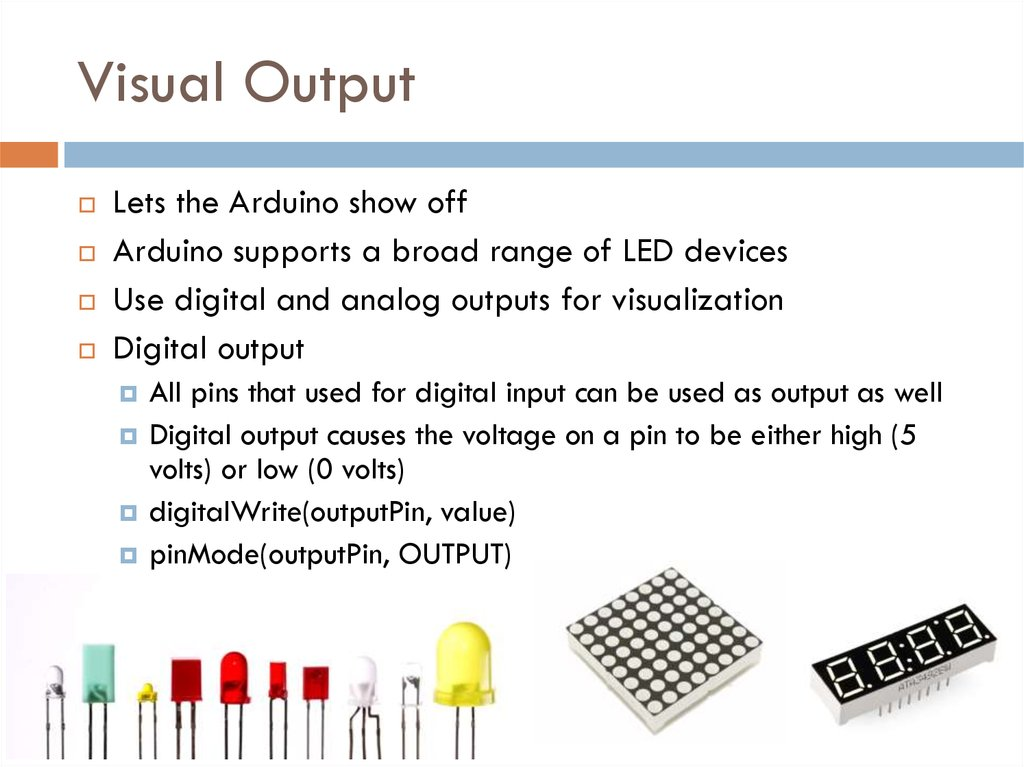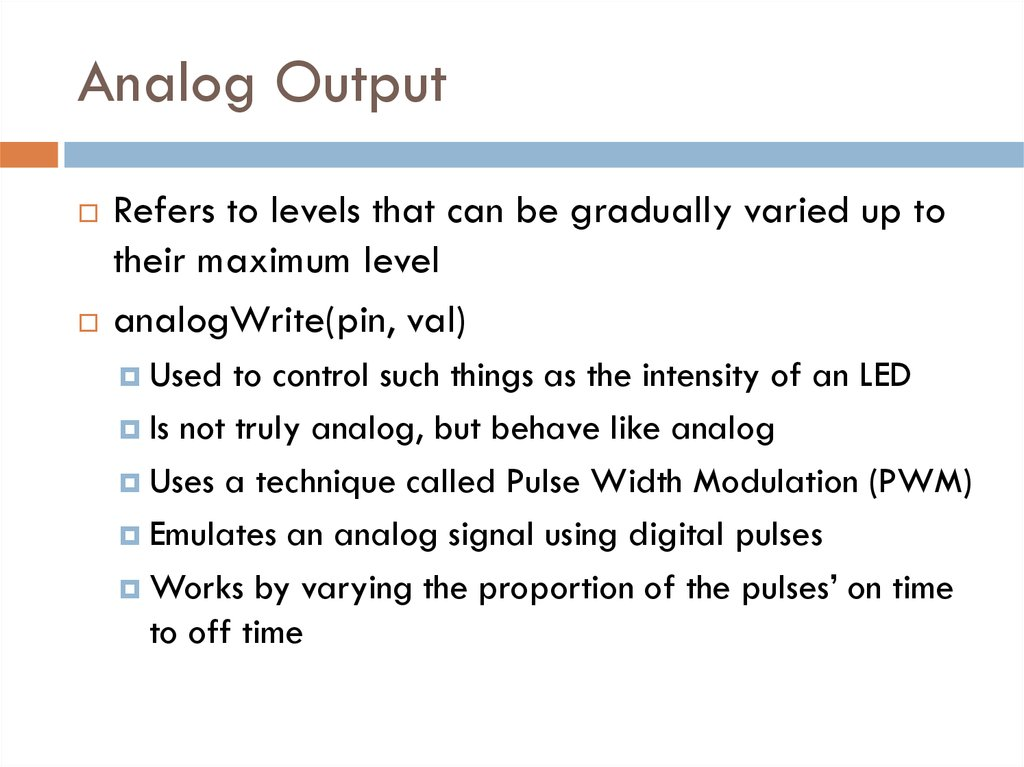# Visual Output

## 1. Implementing IoE

IMPLEMENTING IOE
Week 6
Assist. Prof. Rassim Suliyev - SDU 2017

## 2. Visual Output

Lets the Arduino show off
Arduino supports a broad range of LED devices
Use digital and analog outputs for visualization
Digital output
All pins that used for digital input can be used as output as well
Digital output causes the voltage on a pin to be either high (5
volts) or low (0 volts)
digitalWrite(outputPin, value)
pinMode(outputPin, OUTPUT)

## 3. Analog Output

Refers to levels that can be gradually varied up to
their maximum level
analogWrite(pin, val)
Used
to control such things as the intensity of an LED
Is not truly analog, but behave like analog
Uses a technique called Pulse Width Modulation (PWM)
Emulates an analog signal using digital pulses
Works by varying the proportion of the pulses’ on time
to off time

## 4. Pulse Width Modulation

More commonly called “PWM”
Computers can’t output analog voltages
Only
digital voltages (0 volts or 5 volts)
But you can fake it
if
you average a digital signal flipping between two
voltages
For example...

## 5. PWM

Output voltage is averaged from on vs. off time
output_voltage
= (on_time / off_time) * max_voltage

## 6. PWM

Used everywhere
Lamp
dimmers
Motor speed control
Power supplies
Noise making
Three characteristics of PWM signals
Pulse
width range (min/max)
Pulse period (= 1/pulses per second)
Voltage levels (0-5V, for instance)

## 7. Arduino PWM

Arduino has built-in PWM
Use analogWrite(pin,value)
pin: the pin to write to.
value: the duty cycle: between 0 (always off) and 255 (always
on).
It operates at a high, fixed frequency
On UNO they are pins 3, 5, 6, 9, 10, 11
490HZ for most pins (except pins 5 and 6 which run at 980HZ)
Great for LEDs and motors
Uses built-in PWM circuits of the ATmega8 chip
No software needed

## 8. Arduino PWM

Higher level
output is emulated
with pulses that
are on more than
they are off
Pulses are
repeated quickly
enough
almost 500 times
per second on
Arduino
pulsing cannot be
detected by
human senses

## 9. LED specifications

LED is a semiconductor device (diode)
The device emits light (photons) when
Two leads, an anode and a cathode
Vanode > Vcathode + forward voltage
Anode is usually the longer lead and flat spot on the housing
indicates the cathode
LED color and forward voltage depend on the construction
of the diode
Typical red LED has a forward voltage of around 1.8 volts
Limit the current with a resistor, or the LED will burn out

## 10. Consult an LED data sheet

Arduino pins can supply up to 40 mA of current
This is plenty for a typical medium intensity LED, but not enough to drive the higher brightness
LEDs or multiple LEDs connected to a single pin

## 11. Adjusting the Brightness of an LED

Connect each LED to an analog (PWM) output
const int firstLed = 3;
const int secondLed = 5;
const int thirdLed = 6;
int brightness = 0;
int increment = 1;
void setup(){
//for analogWrite no need
//to declare as OUTPUT
}
void loop(){
if(brightness > 254){
increment = -1; // count down after reaching 254
}else if(brightness < 1){
increment = 1; // count up after dropping back down to 0
}
brightness = brightness + increment; // increment (or decrement sign is minus)
// write the brightness value to the LEDs
analogWrite(firstLed, brightness);
analogWrite(secondLed, brightness);
analogWrite(thirdLed, brightness );
delay(10); // 10ms for each step change means 2.55 secs to fade up or down
}

## 12. Driving High-Power LEDs

Arduino can handle current up to 40 mA per pin
Use a transistor to switch on and off the current
Arrow indicates a +V power source
+5V power pin can supply up to 400 mA or so
If an external power supply is used, remember to connect the ground of
the external supply to the Arduino ground
Current flows from collector to
emitter when transistor is ON
Turn ON transistor by writing HIGH
to appropriate pin
Resistor between the pin and the
transistor base prevents huge current
flow (1K-5mA)
Voltage drop of transistor ~ 0.7V
(collector-emitter saturation voltage)

## 13. How to Exceed 40 mA per Pin

Connect multiple pins in parallel to increase current
beyond the 40 mA
Don’t try to use a single resistor to connect the two
pins
This technique can also be used to source current
It does not work with analogWrite

## 14. Adjusting the Color of an LED

RGB LEDs have red, green, and blue elements in a
single package
common
anode or common cathode

## 15. Adjusting the Color of an LED

const int redPin = 3; // choose the pin for each of the LEDs
const int greenPin = 5;
const int bluePin = 6;
int R, G, B, seg, i; // the Red Green and Blue color components
void setup(){
R = G = B = seg = i = 0;
}
void loop(){
if(i > (255 * 8 - 1)) i = 0;
switch(i / 255){
case 0: R++; break;
case 1: G++; break;
case 2: R--; break;
case 3: B++; break;
case 4: R++; break;
case 5: G--; break;
case 6: R--; break;
case 7: B--; break;
}
analogWrite(redPin, 255 - R);
analogWrite(greenPin, 255 - G);
analogWrite(bluePin, 255 - B);
delay(3);
i++;
}

## 16. Driving a 7-Segment LED Display

Contains 8 LEDs (including Decimal Point indicator)
Common Anode & Common Cathode

## 17. Driving a 7-Segment LED Display

const byte numeral = {
{1,1,1,1,1,1,0,0}, // 0
{0,1,1,0,0,0,0,0}, // 1
{1,1,0,1,1,0,1,0}, // 2
{1,1,1,1,0,0,1,0}, // 3
{0,1,1,0,0,1,1,0}, // 4
{1,0,1,1,0,1,1,0}, // 5
{0,0,1,1,1,1,1,0}, // 6
{1,1,1,0,0,0,0,0}, // 7
{1,1,1,1,1,1,1,0}, // 8
{1,1,1,0,0,1,1,0} // 9
}; //A,B,C,D,E,F,G,dp
const int segmentPins = {2,3,4,6,7,8,9,5}; // A,B,C,D,E,F,G,dp
void setup(){
for(int i=0; i < 8; i++)
pinMode(segmentPins[i], OUTPUT); // set segment and DP pins to output
}
void loop(){
for(int i=0; i < 10; i++){
showDigit(i); delay(1000);
}
}
void showDigit(int number){
if( number >= 0 && number <= 9){
for(int segment = 0; segment < 8; segment++){
int isBitSet = ! numeral[number][segment];; // remove ! sign if common cathode display
digitalWrite(segmentPins[segment], isBitSet);
}
}
}

## 18. Multidigit, 7-Segment: Multiplexing

Corresponding segments from each digit are
connected together

## 19. Multidigit, 7-Segment: Multiplexing

const byte numeral
{1,1,1,1,1,1,0,0}, //
{0,1,1,0,0,0,0,0}, //
{1,1,0,1,1,0,1,0}, //
{1,1,1,1,0,0,1,0}, //
{0,1,1,0,0,1,1,0}, //
{1,0,1,1,0,1,1,0}, //
{0,0,1,1,1,1,1,0}, //
{1,1,1,0,0,0,0,0}, //
{1,1,1,1,1,1,1,0}, //
{1,1,1,0,0,1,1,0} //
}; //A,B,C,D,E,F,G,dp
= {
0
1
2
3
4
5
6
7
8
9
const int segmentPins = {9,2,3,5,6,8,7,4};
// A-11,B-7,C-4,D-2,E-1,F-10,G-5,dp-3
const int digitPins = {13,12,11,10};
// DIG4-6,DIG3-8,DIG2-9,DIG1-12
unsigned long count = 0;
void setup(){
for(int i=0; i < 8; i++)
pinMode(segmentPins[i], OUTPUT);
for(int i=0; i < 4; i++)
pinMode(digitPins[i], OUTPUT);
}
void loop(){
if(millis()/1000 > count) count++;
showNumber(count);
}
void showNumber(int num){
for(int i = 0; i < 4; i++){
showDigit(num % 10, i);
num = num / 10;
}
}
void showDigit(int digit, int pos){
if( digit >= 0 && digit <= 9){
for(int segment = 0; segment < 8; segment++)
digitalWrite(segmentPins[segment], numeral[digit][segment]);
digitalWrite(digitPins[pos],LOW);
delayMicroseconds(300);
digitalWrite(digitPins[pos],HIGH);
}
}

## 20. Multiplexing

To control many LEDs use a technique called multiplexing
Multiplexing is switching groups of LEDs in sequence
Scanning through the LEDs quickly enough
Usually arranged in rows or columns
Creates the impression that the lights remain on
Through the phenomenon of persistence of vision
Charlieplexing uses multiplexing along with the fact that
LEDs have polarity
They only illuminate when the anode is more positive than the
cathode
Switch between two LEDs by reversing the polarity

## 21. Controlling an LED Matrix

8X8 LED matrix contains 64 LEDs
Anodes connected in rows and cathodes in columns
Resistors must be chosen so that max. current
through a pin does not exceed 40 mA
8 LEDs in column => 5mA for each => 680ohms

## 22. Lighting Each Pixel of LED Matrix

const int columnPins[] = { 2, 3, 4, 5, 6, 7, 8, 9};
const int rowPins[] = { 10,11,12,14,15,16,17,18};
int pixel = 0; // 0 to 63 LEDs in the matrix
int columnLevel = 0; // pixel value converted into LED column
int rowLevel = 0; // pixel value converted into LED row
void setup() {
for (int i = 0; i < 8; i++){
pinMode(columnPins[i], OUTPUT); // make all the LED pins outputs
digitalWrite(columnPins[i], HIGH);
pinMode(rowPins[i], OUTPUT);
digitalWrite(rowPins[i], LOW);
}
}
void loop() {
pixel = pixel + 1;
if(pixel > 63) pixel = 0;
columnLevel = pixel / 8; // map to the number of columns
rowLevel = pixel % 8; // get the fractional value
digitalWrite(columnPins[columnLevel], LOW); // connect this column to Ground
digitalWrite(rowPins[rowLevel], HIGH);
delay(100);
digitalWrite(rowPins[rowLevel], LOW); // turn off LED
digitalWrite(columnPins[columnLevel], HIGH);
}

## 23. Displaying Images on an LED Matrix

byte bigHeart[] = {
B01100110,
B11111111,
B11111111,
B11111111,
B01111110,
B00111100,
B00011000,
B00000000};
byte smallHeart[] = {
B00000000,
B00000000,
B00010100,
B00111110,
B00111110,
B00011100,
B00001000,
B00000000};
const int columnPins[] = { 2, 3, 4, 5, 6, 7, 8, 9};
const int rowPins[] = { 10,11,12,14,15,16,17,18};
void setup() {
for (int i = 0; i < 8; i++){
pinMode(rowPins[i], OUTPUT); // make all the LED pins outputs
pinMode(columnPins[i], OUTPUT);
digitalWrite(columnPins[i], HIGH); // disconnect column pins
}
}
void loop() {
show(smallHeart, 800); // show the small heart image for 100 ms
show(bigHeart, 800); // followed by the big heart for 200ms
delay(400); // show nothing between beats
}
void show( byte * image, unsigned long duration){
unsigned long start = millis(); // begin timing the animation
while (start + duration > millis()){ // loop until duration passed
for(int row = 0; row < 8; row++){
digitalWrite(rowPins[row], HIGH); // connect row to +5 volts
for(int column = 0; column < 8; column++){
if(pixel == 1) digitalWrite(columnPins[column], LOW);
delayMicroseconds(300); // a small delay for each LED
digitalWrite(columnPins[column], HIGH);
}
digitalWrite(rowPins[row], LOW); // disconnect LEDs
}
}
}

## 24. Controlling LEDs: Charlieplexing

Charlieplexing - increases the number of LEDs that
can be driven by a group of pins
Based on the fact that LEDs only turn on when
anode more positive than the cathode

## 25. Controlling LEDs: Charlieplexing

byte pins[] = {2,3,4}; // the pins that are connected to LEDs
const int NUMBER_OF_PINS = sizeof(pins)/ sizeof(pins);
const int NUMBER_OF_LEDS = NUMBER_OF_PINS * (NUMBER_OF_PINS-1);
byte pairs[NUMBER_OF_LEDS/2] = { {0,1}, {1,2}, {0,2} }; // maps pins to LEDs
void setup(){
}
void loop(){
for(int i=0; i < NUMBER_OF_LEDS; i++){
lightLed(i); // light each LED in turn
delay(1000);
}
}
void lightLed(int led){
int indexA = pairs[led/2];
int indexB = pairs[led/2];
int pinA = pins[indexA];
int pinB = pins[indexB];
for(int i=0; i < NUMBER_OF_PINS; i++)
if( pins[i] != pinA && pins[i] != pinB){ // if this pin is not one of our pins
pinMode(pins[i], INPUT); // set the mode to input
digitalWrite(pins[i],LOW); // make sure pull-up is off
}
pinMode(pinA, OUTPUT); pinMode(pinB, OUTPUT);
if( led % 2 == 0){
digitalWrite(pinA,LOW); digitalWrite(pinB,HIGH);
}else{
digitalWrite(pinB,LOW); digitalWrite(pinA,HIGH);
}
}